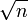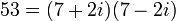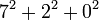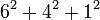53

Summary

Factorization

The number 53 is a prime number.

Properties and families

Property or family Parameter values First few numbers Proof of satisfaction/membership/containment
prime number 2, 3, 5, 7, 11, 13, 17, 19, 23, 29, 31, 37, 41, 43, 47, 53, 59, 61, 67, 71, 73, 79, 83, 89, 97, [SHOW MORE]View list on OEIS A natural numberis prime if and only ifis not divisible by any prime less than or equal to. In this case, sinceis between 7 and 8, verifying primality requires checking that 53 is not divisible by any prime up to 7, i.e., it is not divisible by 2, 3, 5, or 7.
regular prime 3, 5, 7, 11, 13, 17, 19, 23, 29, 31, 41, 43, 47, 53, 61, [SHOW MORE]View list on OEIS

Waring representations

Sums of squares

Item Value
unique (up to plus/minus and ordering) representation as sum of two squares. Note that existence and uniqueness both follow from it being a prime that is 1 mod 4.
This also corresponds to the factorizationin the ring of Gaussian integers.
representations as sum of three squares (up to ordering and plus/minus equivalence)Prime-generating polynomials

Below are some polynomials that give prime numbers for small input values, which give the value 53 for suitable input choice.

Polynomial Degree Some values for which it generates primes Input valueat which it generates 532 all numbers 1-10, because 11 is one of the lucky numbers of Euler. 72 all numbers 1-40, because 41 is one of the lucky numbers of Euler. 4